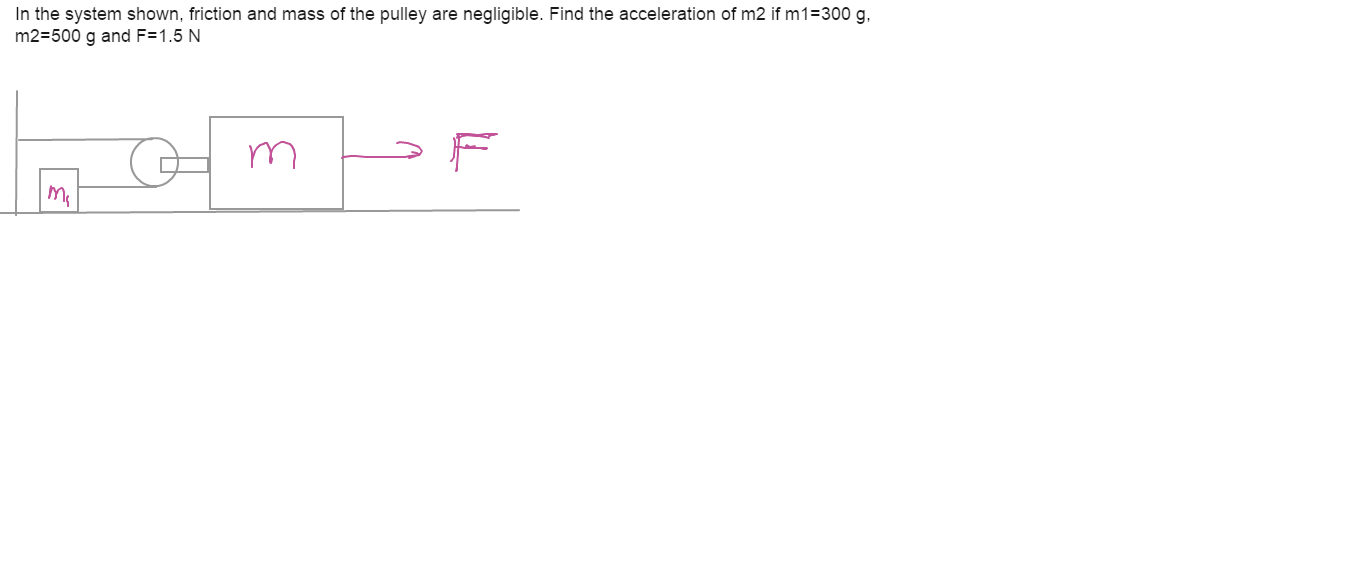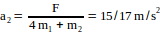# see attach8 years ago
p { margin-bottom: 0.25cm; line-height: 120%; }

Let

T = tension in the string connected to m1,

a1 = acceleration of mass m1

a2 = acceleration of mass m2

Then from constraint equation for the string, we can write a1 = 2a2 eq(1).

From free body diagram for m1, T = m1a1 eq(2).

From free body diagram for m2, F – 2T = m2a2 eq(3)

Solving above equations, we get